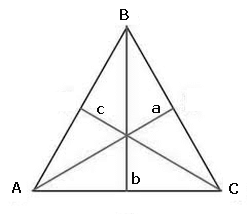### Equilateral triangle

Equilateral triangle is a polygon composed by 3 equal sides and equal all interior angles. The polygon is a closed plane figure composed by a non specified number of straight line segments that join in pairs at their endpoints. So, the equilateral triangle is a polygon with three equal sides.
Read full text in Equilateral triangle.
To calculate equilateral triangle enter the requested information, using the point as a decimal separator. Ex 1,350.64 enter: 1350.64; the results will be shown after a click on CalculateEquilateral Triangle Decimal places: Side length:     Height : Perimeter: Area: Incircle radius: Excircle radius: Angle (A=B=C): Nota:

Note: The accuracy of the calculator and its applicability to particular cases is not guaranteed.